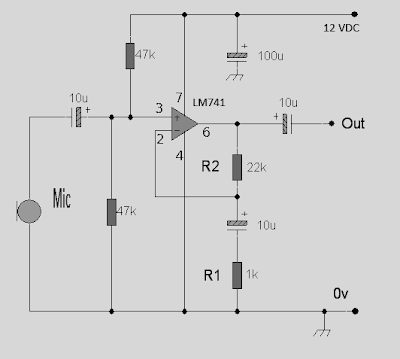## Monday, January 25, 2010

### Rangkaian Op-Amp (LM741) Pre-Amp Mic

Op-Amp Pre-Amp Mic

This is the circuit of Op-Amp Microphone Preamplifier using a single power supply, this circuit suitable for dynamic or electret microphones. Nothing too special here. The Schematic is shown using a dynamic microphone, for use with an electret a pair of suitable biasing resistor is required to power the electret microphone.Skema rangkaian op-amp pre-amp mic

Note:
• use a capacitor with voltage 25volt or more
• so that the sound produced maximal use supplay good voltage, with output of 18 volts max
• If the desired strengthening of the different, you can change the value of R1 or R2

The design is a standard non-inverting design, the input is applied to the non-inverting input of the op-amp, which is pin 3 in most cases. The input impedance is 23.5k, the overall voltage gain is determined by R2 and R1according to the following formula:

Vo = (R2 / R1) + 1

With the values of R2 and R1 on the diagram of the voltage gain (for mid band, 1kHz) is approximately 23x or 27.2dB.

Pinning IC Op-Amp LM741Skema Rangkaian Elektronika